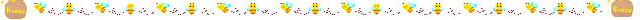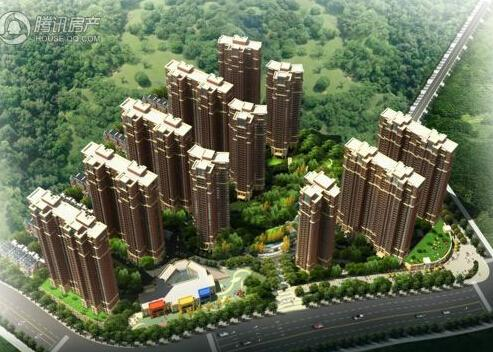# 定制购房：高铁新城亲民价学位房 享受12年一站教育

080平*6000元/平=48万；首付=14.4万；月供=2387

122平*6000元/平=73.2万；首付=21.96万；月供=364190平*7000元/平=63万；首付=18.9万；月供=3134

122平*7000元/平=85.4万；首付=25.62万；月供=4248

89平*6800元/平=63.2万；首付=18.15万；月供=3010

122平*6800元/平=82.96万；首付=24.88万；月供=412756平*6300元/平=35.28万；首付=10.56万；月供=1755

156平*6300元/平=98.28万；首付=29.48万；月供=4889

77平*6000元/平=46.2万；首付=13.86万；月供=2298

143平*6000元/平=85.8万；首付=25.74万；月供=4268

[责任编辑：wycathy]